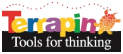Terrapin Resources

# Introductory Lessons

## Lesson 1: What is One Distance of Bee-Bot?

### Overview

Students will determine the distance of 1 unit of Bee-Bot. This will be helpful when using the Bee-Bot and solving future challenges.

• Estimation
• Distance
• Measurement

### Materials Needed

• Bee-Bot
• String or other resource for measuring such as yarn or ribbon.
• Marker
• Scissors

### Activity

Place the Bee-Bot on the floor.

1. Have a student place one end of string next to the front of the Bee-Bot.
2. Have a student press the forward arrow one time and then Go.
3. The Bee-Bot will move forward 1 unit.
4. Mark the string where the front of the Bee-Bot stopped.
5. Cut the String where marked. This represents the distance of one unit of Bee-Bot.

### Extension

• Have students create a name for the unit of measurement.
• Compare the string to a ruler and see how many inches/centimeters one unit of Bee- Bot represents.
• Estimate how many units it will take to move Bee-Bot to a designated location in the classroom. Try it and see if you were right, need more, or less.

## Lesson 2: Getting to Know You

### Overview

Students will apply units of measurement to move the Bee-Bot to another location.

• Estimation
• Distance
• Measurement
• Direction

• Bee-Bot

### Activity

1. Have students sit in a circle.
2. One student picks another student sitting in the circle and estimates how many units it will take Bee-Bot to reach the selected student.
3. The student will estimate, test, and adjust if necessary.
4. Once the Bee-Bot reaches the student, have that student answer a question before selecting another student to send the Bee-Bot to. They could perhaps introduce themselves if it is the beginning of the school year, their favorite activity, a book they recently read they would recommend, etc. There are infinite ways you can incorporate questions from current units of study.

### Extension

• Addition problems: Write the student’s initial estimate on a chalkboard, dry erase board, somewhere where all can see. For example if a student estimates forward 5 write 5. If the estimate is too short put a plus sign next to the 5, creating an addition problem and “add” the additional units need to reach the desired location. Place the Bee-Bot back where it began, Enter the additional units and select Go. (Bee-Bot remembers the previous entry) OR Clear Bee-Bots memory by selecting the Clear (x) key and enter the new estimated total.
• Subtraction problems: Apply the same concept for subtraction. If the Bee-Bot goes too far, students can use the back button to take away steps. Make sure you place the Bee-Bot back where it started.

Don’t forget the Bee-Bot holds all previous commands entered, and adds new ones onto the end. You can delete previous commands by selecting the X (clear) button.